# RD Sharma Solutions For Class 12 Maths Exercise 18.3 Chapter 18 Maxima and Minima

RD Sharma Solutions for Class 12 Maths Exercise 18.3 Chapter 18 Maxima and Minima is provided here. Exercise 18.3 of Chapter 18 deals with the problems based on the higher-order derivative test. To improve conceptual knowledge among students, we at BYJU’S have a set of experts designing the solutions, as per CBSE guidelines.

The PDF of RD Sharma Solutions for Class 12 Maths Exercise 18.3 of Chapter 18 Maxima and Minima is provided here. Learners can easily access and download the solutions as their study material. Some of the important topics of this exercise are listed below.

• Higher-order derivative test
• Theorem and algorithm based on higher derivative test
• Point of inflection
• Point of inflexion
• Properties of maxima and minima

## Download the PDF of RD Sharma Solutions For Class 12 Chapter 18 – Maxima and Minima Exercise 18.3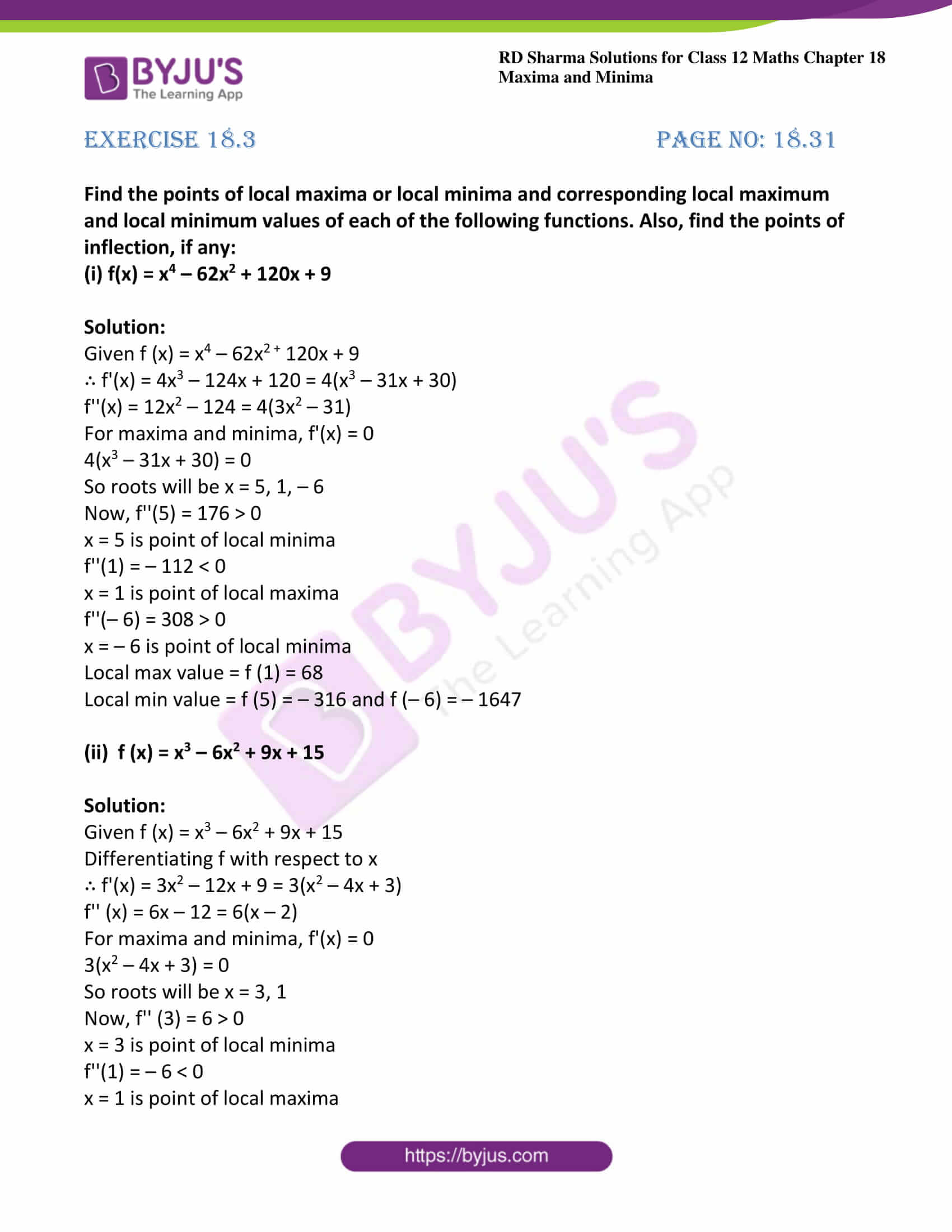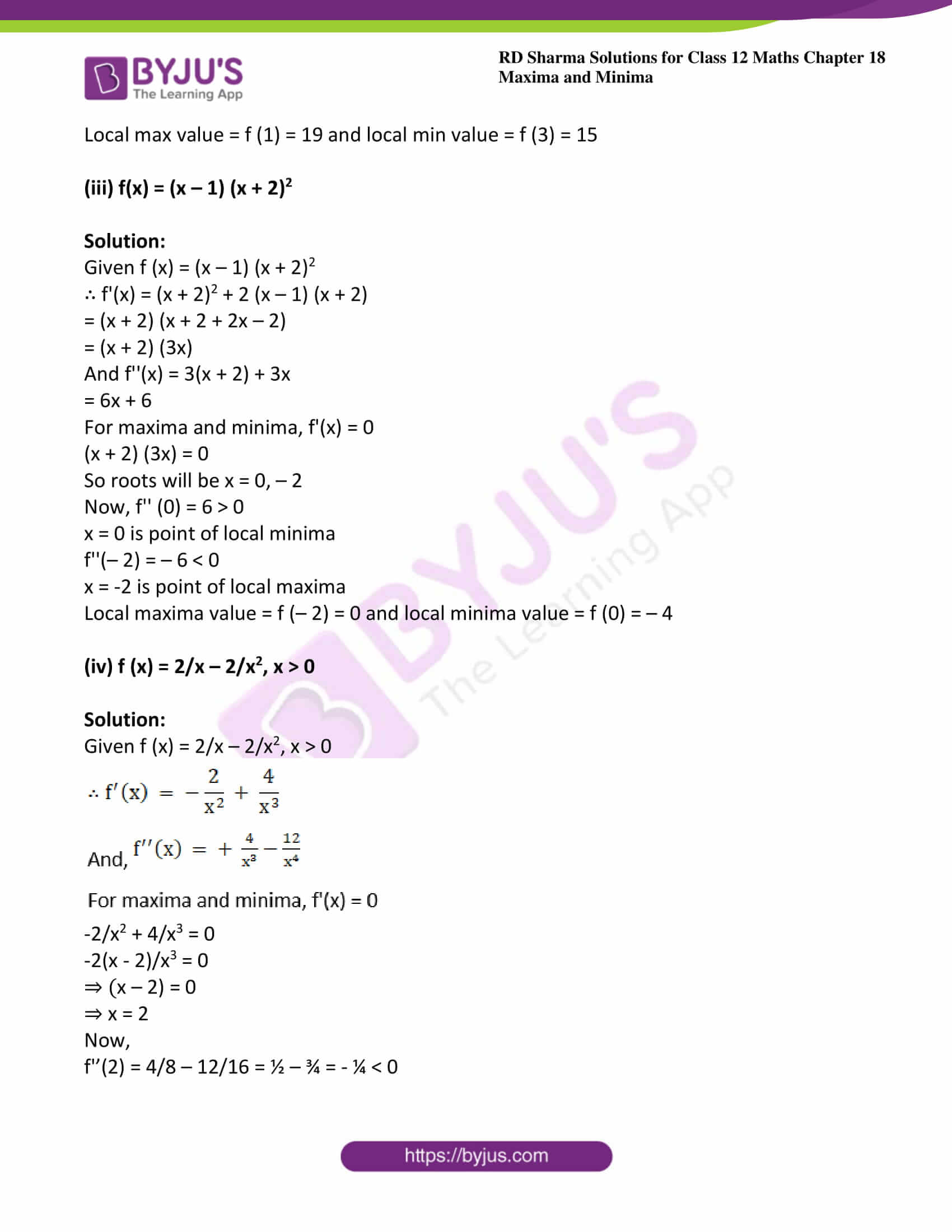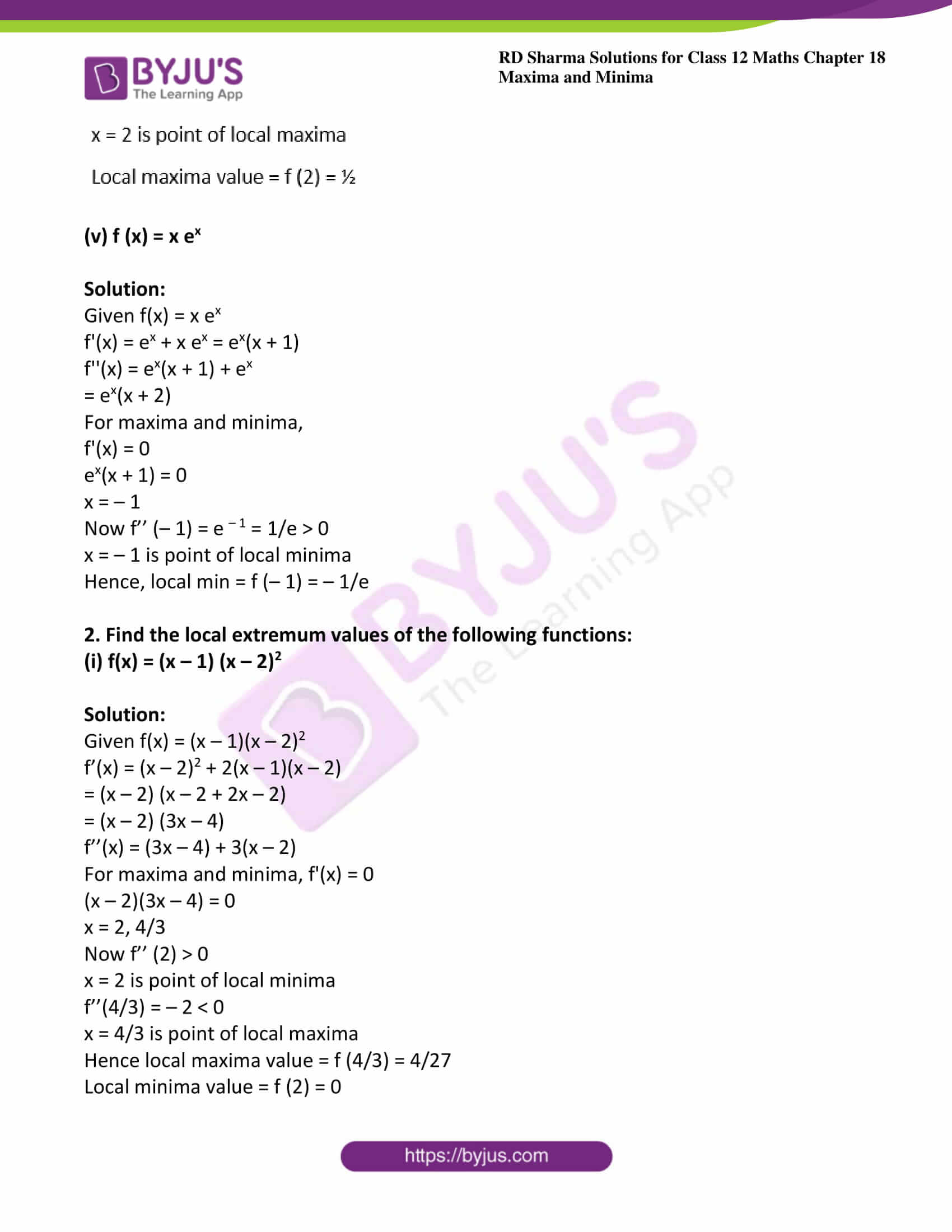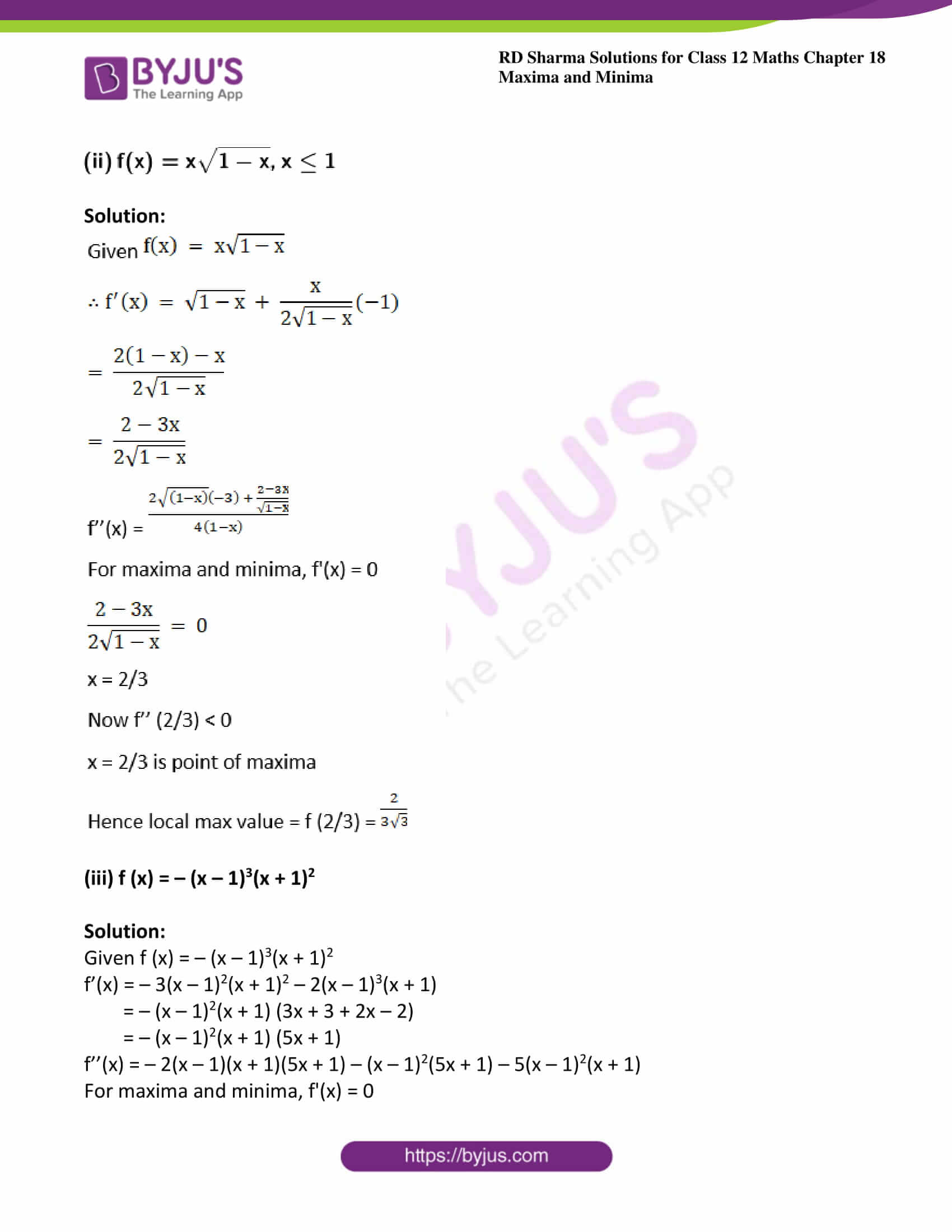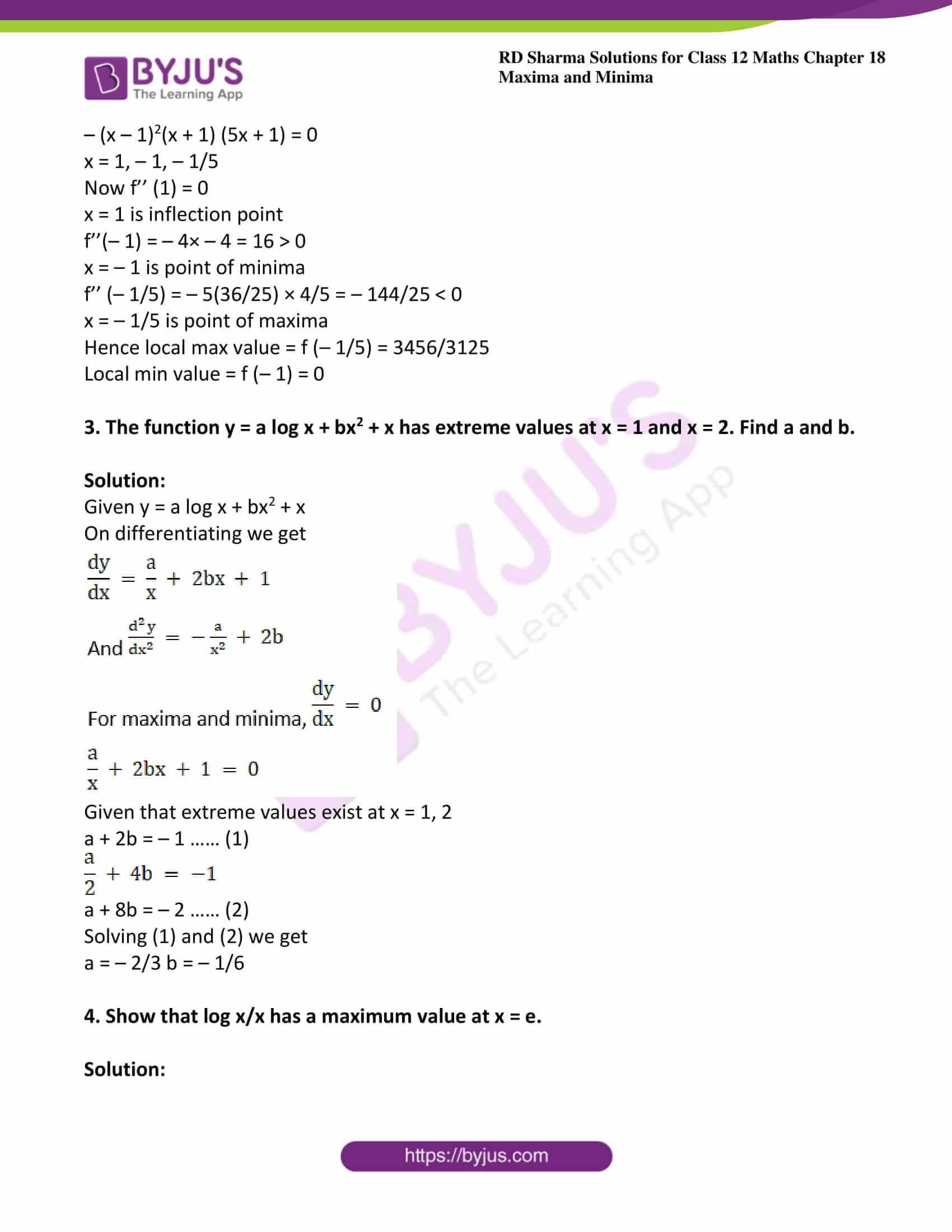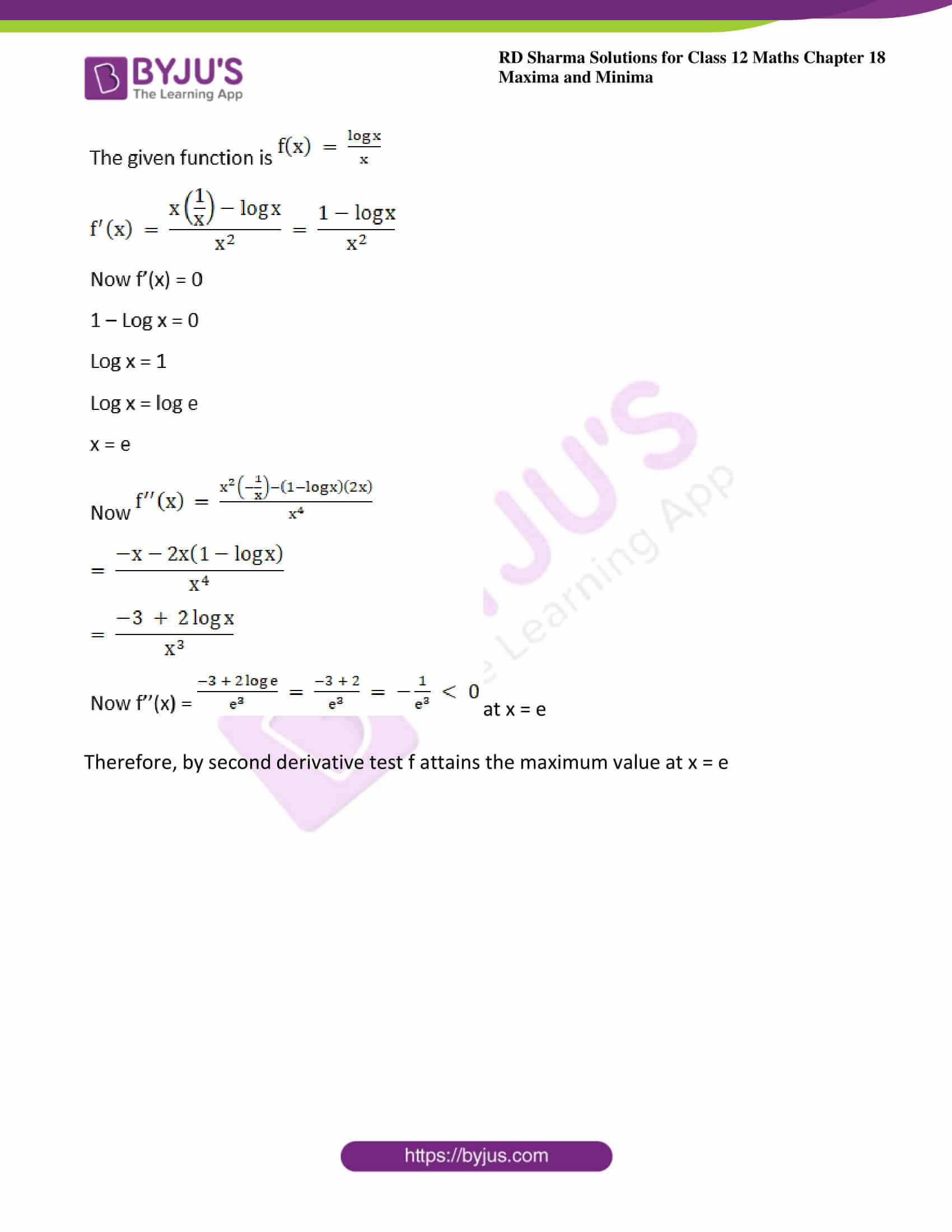### Access other exercises of RD Sharma Solutions For Class 12 Chapter 18 – Maxima and Minima

Exercise 18.1 Solutions

Exercise 18.2 Solutions

Exercise 18.4 Solutions

Exercise 18.5 Solutions

### Exercise 18.3 Page No: 18.31

Find the points of local maxima or local minima and corresponding local maximum and local minimum values of each of the following functions. Also, find the points of inflection, if any:

(i) f(x) = x4 – 62x2 + 120x + 9

Solution:

Given f (x) = x4 – 62x2 + 120x + 9

∴ f'(x) = 4x3 – 124x + 120 = 4(x3 – 31x + 30)

f”(x) = 12x2 – 124 = 4(3x2 – 31)

For maxima and minima, f'(x) = 0

4(x3 – 31x + 30) = 0

So roots will be x = 5, 1, – 6

Now, f”(5) = 176 > 0

x = 5 is point of local minima

f”(1) = – 112 < 0

x = 1 is point of local maxima

f”(– 6) = 308 > 0

x = – 6 is point of local minima

Local max value = f (1) = 68

Local min value = f (5) = – 316 and f (– 6) = – 1647

(ii) f (x) = x3 – 6x2 + 9x + 15

Solution:

Given f (x) = x3 – 6x2 + 9x + 15

Differentiating f with respect to x

∴ f'(x) = 3x2 – 12x + 9 = 3(x2 – 4x + 3)

f” (x) = 6x – 12 = 6(x – 2)

For maxima and minima, f'(x) = 0

3(x2 – 4x + 3) = 0

So roots will be x = 3, 1

Now, f” (3) = 6 > 0

x = 3 is point of local minima

f”(1) = – 6 < 0

x = 1 is point of local maxima

Local max value = f (1) = 19 and local min value = f (3) = 15

(iii) f(x) = (x – 1) (x + 2)2

Solution:

Given f (x) = (x – 1) (x + 2)2

∴ f'(x) = (x + 2)2 + 2 (x – 1) (x + 2)

= (x + 2) (x + 2 + 2x – 2)

= (x + 2) (3x)

And f”(x) = 3(x + 2) + 3x

= 6x + 6

For maxima and minima, f'(x) = 0

(x + 2) (3x) = 0

So roots will be x = 0, – 2

Now, f” (0) = 6 > 0

x = 0 is point of local minima

f”(– 2) = – 6 < 0

x = -2 is point of local maxima

Local maxima value = f (– 2) = 0 and local minima value = f (0) = – 4

(iv) f (x) = 2/x – 2/x2, x > 0

Solution:

Given f (x) = 2/x – 2/x2, x > 0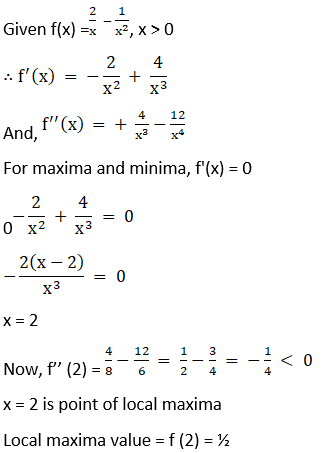-2/x2 + 4/x3 = 0

-2(x – 2)/x3 = 0

⇒ (x – 2) = 0

⇒ x = 2

Now,

f’’(2) = 4/8 – 12/16 = ½ – ¾ = – ¼ < 0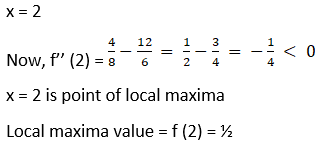(v) f (x) = x ex

Solution:

Given f(x) = x ex

f'(x) = ex + x ex = ex(x + 1)

f”(x) = ex(x + 1) + ex

= ex(x + 2)

For maxima and minima,

f'(x) = 0

ex(x + 1) = 0

x = – 1

Now f’’ (– 1) = e – 1 = 1/e > 0

x = – 1 is point of local minima

Hence, local min = f (– 1) = – 1/e

2. Find the local extremum values of the following functions:
(i) f(x) = (x – 1) (x – 2)2

Solution:

Given f(x) = (x – 1)(x – 2)2

f’(x) = (x – 2)2 + 2(x – 1)(x – 2)

= (x – 2) (x – 2 + 2x – 2)

= (x – 2) (3x – 4)

f’’(x) = (3x – 4) + 3(x – 2)

For maxima and minima, f'(x) = 0

(x – 2)(3x – 4) = 0

x = 2, 4/3

Now f’’ (2) > 0

x = 2 is point of local minima

f’’(4/3) = – 2 < 0

x = 4/3 is point of local maxima

Hence local maxima value = f (4/3) = 4/27

Local minima value = f (2) = 0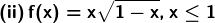Solution: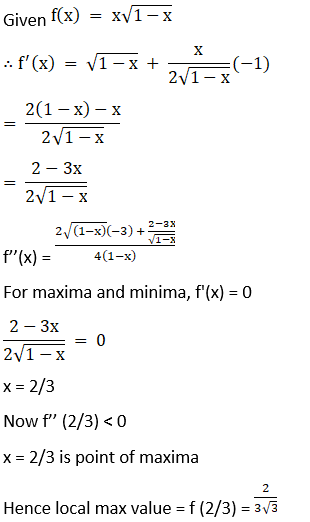(iii) f (x) = – (x – 1)3(x + 1)2

Solution:

Given f (x) = – (x – 1)3(x + 1)2

f’(x) = – 3(x – 1)2(x + 1)2 – 2(x – 1)3(x + 1)

= – (x – 1)2(x + 1) (3x + 3 + 2x – 2)

= – (x – 1)2(x + 1) (5x + 1)

f’’(x) = – 2(x – 1)(x + 1)(5x + 1) – (x – 1)2(5x + 1) – 5(x – 1)2(x + 1)

For maxima and minima, f'(x) = 0

– (x – 1)2(x + 1) (5x + 1) = 0

x = 1, – 1, – 1/5

Now f’’ (1) = 0

x = 1 is inflection point

f’’(– 1) = – 4× – 4 = 16 > 0

x = – 1 is point of minima

f’’ (– 1/5) = – 5(36/25) × 4/5 = – 144/25 < 0

x = – 1/5 is point of maxima

Hence local max value = f (– 1/5) = 3456/3125

Local min value = f (– 1) = 0

3. The function y = a log x + bx2 + x has extreme values at x = 1 and x = 2. Find a and b.

Solution:

Given y = a log x + bx2 + x

On differentiating we get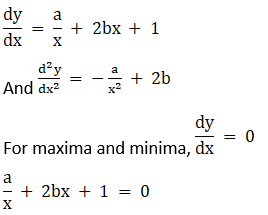Given that extreme values exist at x = 1, 2

a + 2b = – 1 …… (1)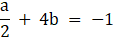a + 8b = – 2 …… (2)

Solving (1) and (2) we get

a = – 2/3 b = – 1/6

4. Show that log x/x has a maximum value at x = e.

Solution: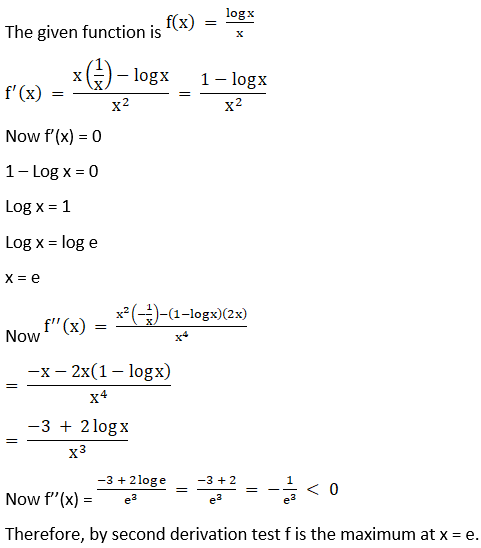at x = e

Therefore, by second derivative test f attains the maximum value at x = e